## SIGNAL PROCESSING Laboratory #3

#### M. Kociński, P. Strumiłło, A. Borowska-Terka Medical Electronics Division Institute of Electronics

In :
import funkcje_do_lab3 as f3
f3.hide()

Out:
The raw code for this IPython notebook is by default hidden for easier reading. To toggle on/off the raw code, click here.

PURPOSE:

To learn how to import Python packages and how to load and save text, binary, Matlab and wave files files.

1.Learn four ways of importing Phyton packages inside scripts. Please create and run the following scripts in your Python folder: sinus1.py, sinus2.py, sinus3.py, sinus4.py:

In :
f3.fig(f3.s1)

Out: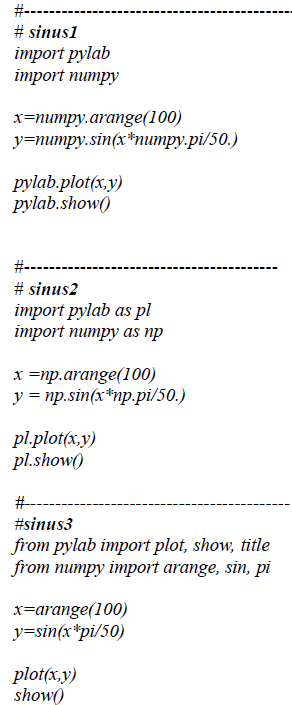In :
f3.fig(f3.s4)

Out: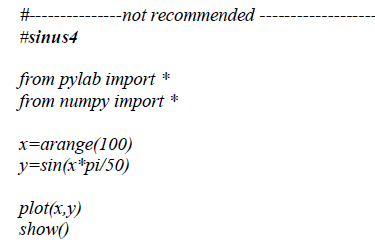EXPECTED RESULTS:

In :
%pylab inline
print ''
print 'sinus1:'
f3.sinus1()

Populating the interactive namespace from numpy and matplotlib

sinus1: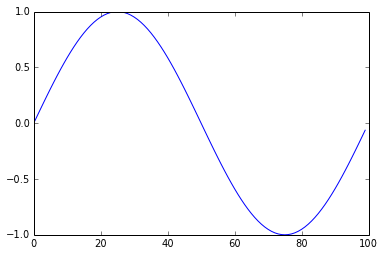In :
print ''
print 'sinus2:'
f3.sinus2()


sinus2:In :
print ''
print 'sinus3:'
f3.sinus3()


sinus3:sinus4 (Not recommended importing!):

In :
from pylab import *
from numpy import *

x=arange(100)
y=sin(x*pi/50)

plot(x,y)
show()2.Loading and saving files in ASCII format (the so called text files)

In :
f3.fig(f3.r)

Out: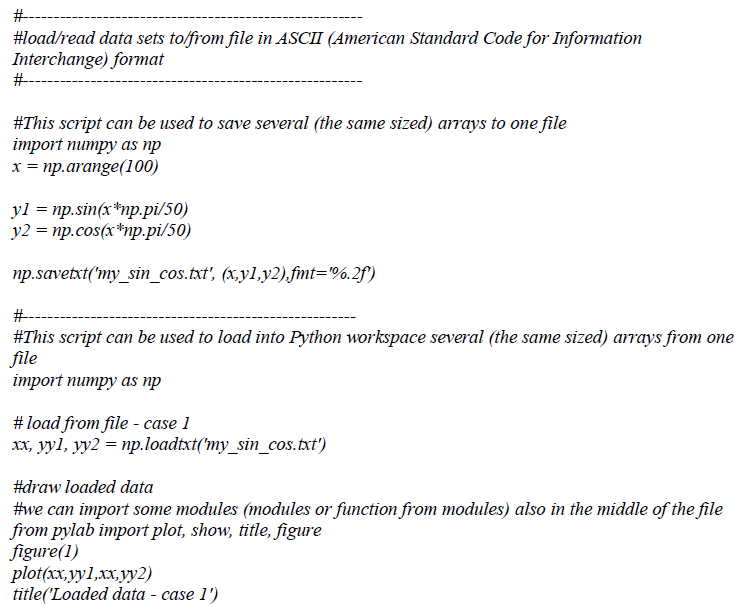For loading/saving files from other directories you need to specify a path to a file, e.g.

In :
import numpy as np
x = np.arange(100)

y1 = np.sin(x*np.pi/50)
y2 = np.cos(x*np.pi/50)

np.savetxt('my_sin_cos.txt', (x,y1,y2),fmt='%.2f')

In :
import os
files = os.listdir('.')   #all files in current directory
print 'Did we save our file my_sin_cos.txt?'
'my_sin_cos.txt' in files #is there our file 'my_sin_cos.txt'?

Did we save our file my_sin_cos.txt?


Out:
True

In :
import numpy as np

# load from file - case 1

#we can import some modules (modules or function from modules) also in the middle of the file
from pylab import plot, show, title, figure
figure(1)
plot(xx,yy1,xx,yy2)
show()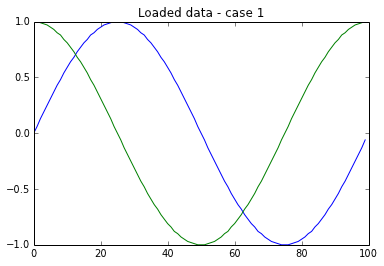3.Loading and saving binary files in Python native formats .npy and .npyz

In :
f3.fig('binary_files.png')

Out: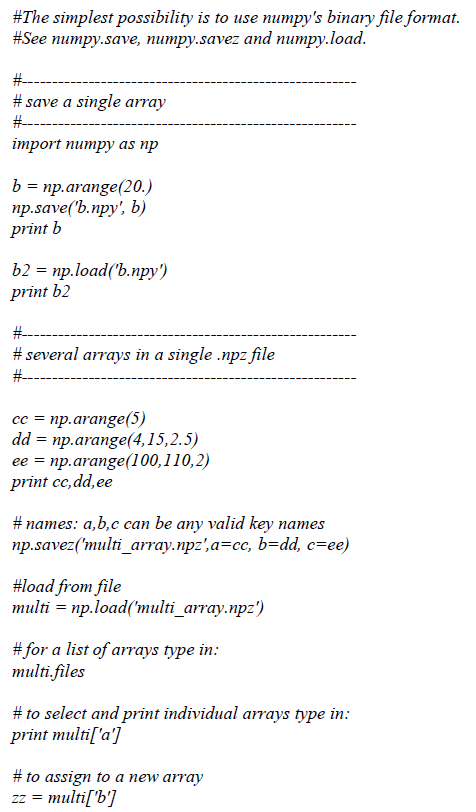In :
import numpy as np

b = np.arange(20.)
np.save('b.npy', b)
print 'The variable b:'
print b

print 'The content of file b2.npy:'
print b2

The variable b:
[  0.   1.   2.   3.   4.   5.   6.   7.   8.   9.  10.  11.  12.  13.  14.
15.  16.  17.  18.  19.]
The content of file b2.npy:
[  0.   1.   2.   3.   4.   5.   6.   7.   8.   9.  10.  11.  12.  13.  14.
15.  16.  17.  18.  19.]


In :
cc = np.arange(5)
dd = np.arange(4,15,2.5)
ee = np.arange(100,110,2)
print 'cc:'
print cc
print 'dd:'
print dd
print 'ee:'
print ee
print ''
print 5*'*'
# names: a,b,c can be any valid key names
np.savez('multi_array.npz',a=cc, b=dd, c=ee)

print 'List of arrays type in:'
print multi.files
print ''

# to select and print individual arrays type in:
print 'Array a:'
print multi['a']
print ''

# to assign to a new array
zz = multi['b']
print 'Array zz:'
print zz

cc:
[0 1 2 3 4]
dd:
[  4.    6.5   9.   11.5  14. ]
ee:
[100 102 104 106 108]

*****
List of arrays type in:
['a', 'c', 'b']

Array a:
[0 1 2 3 4]

Array zz:
[  4.    6.5   9.   11.5  14. ]



In :
f3.fig('matlab_files.png')

Out: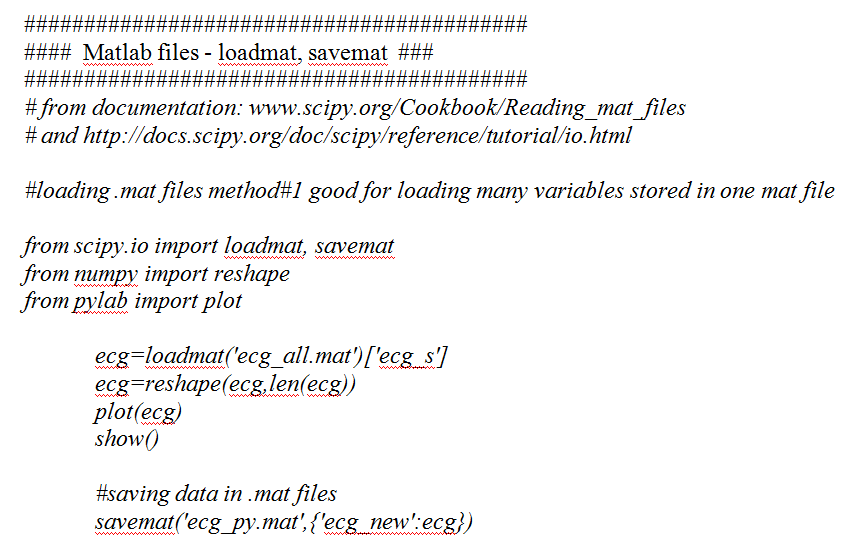In :
from scipy.io import loadmat, savemat
from numpy import reshape
from pylab import plot

ecg=reshape(ecg,len(ecg))
plot(ecg)
show()

#saving data in .mat files
savemat('ecg_py.mat',{'ecg_new':ecg})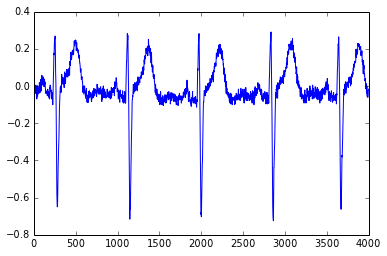In :
f3.fig('play_audio.png')

Out: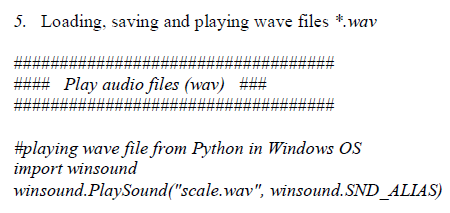In :
import winsound
winsound.PlaySound("scale.wav", winsound.SND_ALIAS)

In :
f3.fig('load_wave.png')

Out: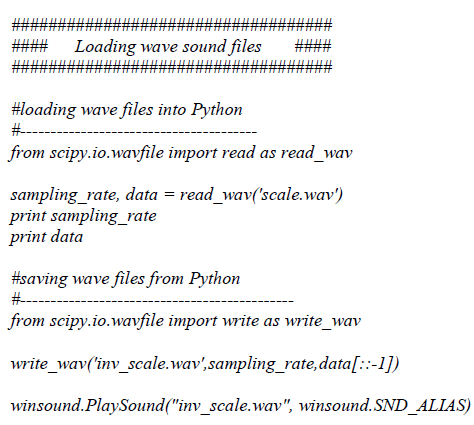EXPECTED RESULTS:

In :
from scipy.io.wavfile import read as read_wav

print sampling_rate
print data

11025
[     0   2970   5876 ..., -19999 -19250 -16986]


In :
from scipy.io.wavfile import write as write_wav

write_wav('inv_scale.wav',sampling_rate,data[::-1])

winsound.PlaySound("inv_scale.wav", winsound.SND_ALIAS)


6.You can work with sounds (signals) interactively.
In this case run the command line of your operating system. Change your current directory to D:/Biomed2014_1.
Type in >>ipython notebook
By selecting icon [New notebook] create new file audio.ipynb
Type the following sequence of commands:

from IPython.display import Audio
import numpy as np
f1 = 320
f2 = 345
rate = 8000.0
L=3

times = np.linspace(0, L, rateL)
signal = np.cos(2
np.pif1times) + np.cos(2np.pif2*times)

Audio(data=signal, rate=rate)

By selecting icon [Run Cell] run your script. Alter frequency values f1, f2 and run script again.

EXPECTED RESULTS:

In :
from IPython.display import Audio
import numpy as np
f1 = 320
f2 = 345
rate = 8000.0
L=3

times = np.linspace(0, L, rate*L)
signal = np.cos(2*np.pi*f1*times) + np.cos(2*np.pi*f2*times)

Audio(data=signal, rate=rate)

Out:
In :